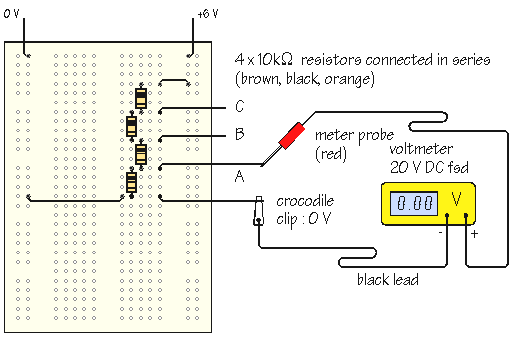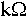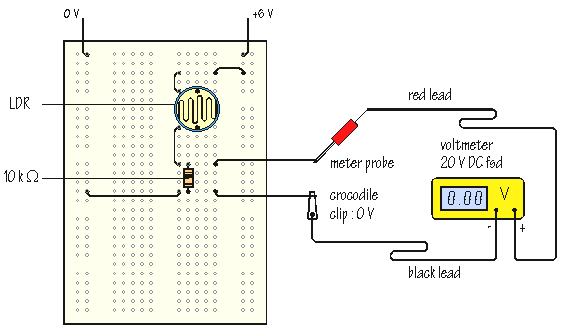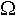Home > Electronic Tutorials > How to use a Multimeter / Using Multimeters > Voltage Measurements Tutorial

How to use a Multimeter / Using Multimeters

# Voltage Measurements Tutorial

## Voltage measurements:Using the multimeter as a voltmeter, measure the power supply voltage and then measure the voltages at points A, B and C.

The four resistors are connected in series, making a chain known as a potential divider, or voltage divider. The total voltage is shared between the four resistors and, allowing for tolerance, each resistor receives an equal share.

Modify the circuit, replacing one or more of the 10resistors with 1or 100values. Are the results as you expect?

The diagram below shows a light sensor circuit built in a similar way:The circuit uses an LDR, or light dependent resistor. The resistance of the LDR changes with illumination. In the dark, the resistance is high, up to 1 Mor more. When light shines on the LDR, the light energy increases the number of charge carriers available to transfer current, and the resistance falls. In bright light, the resistance can be as little as 100.

What happens to the output voltage of the light sensor circuit when you cover the LDR with you hand?

Is the output voltage HIGH or LOW in the dark?